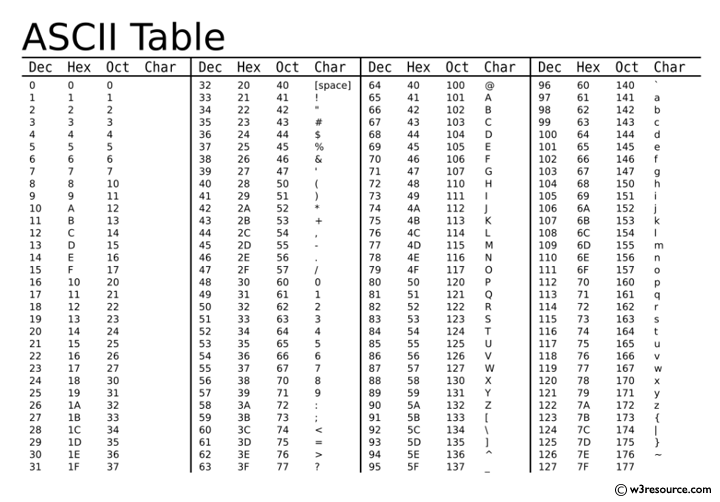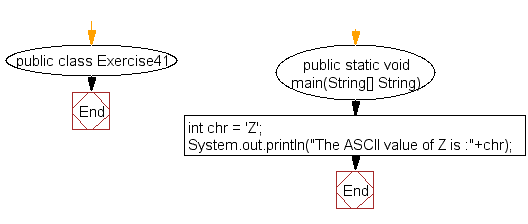﻿ Java exercises: Print the ascii value of a given character - w3resource# Java Exercises: Print the ascii value of a given character

## Java Basic: Exercise-41 with Solution

Write a Java program to print the ascii value of a given character.

Pictorial Presentation:Sample Solution:

Java Code:

``````public class Exercise41 {

public static void main(String[] String) {
int chr = 'Z';
System.out.println("The ASCII value of Z is :"+chr);
}
}
```
```

Sample Output:

```The ASCII value of Z is :90
```

Flowchart:Java Code Editor:

What is the difficulty level of this exercise?

Test your Programming skills with w3resource's quiz.

﻿

## Java: Tips of the Day

getEnumMap

Converts to enum to Map where key is the name and value is Enum itself.

```public static <E extends Enum<E>> Map<String, E> getEnumMap(final Class<E> enumClass) {
return Arrays.stream(enumClass.getEnumConstants())
.collect(Collectors.toMap(Enum::name, Function.identity()));
}
```

Ref: https://bit.ly/3xXcFZt